Next:3. Numerical Discretization MethodsUp:2.2 OxidationPrevious:2.2.2 Point Defect Models

## 2.2.3 Segregation Effects Arising at the Moving Interface

In contrast to the segregation effect described in the previous section where the interface is assumed to be stationary, now an additional effect namely the moving interface due to oxidation phenomena has to be taken into account (Fig. 2.4):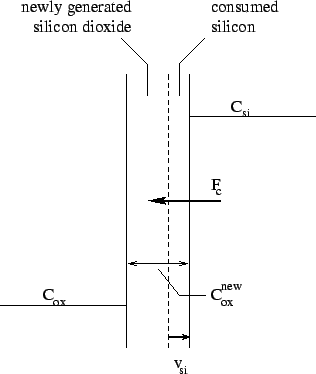• the interface between silicon and silicon dioxide changes its position depending on the velocity term
•  vsi = -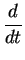dsi (2.43)

where dsi denotes the thickness of silicon

• a part of the silicon layer is consumed by the local oxidation (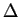dsi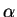dox) and results in a change of the distribution of particles. This mass transport of dopants can be described by a flux Fc proportional to the velocity vsi
Ignoring the mass transport phenomena described in Section 2.1.3, which is equivalent to the conditions Fi = 0, Fsi = 0, and Fox = 0, the concentration Coxnew would be proportional to the interface concentration Csieq
 Coxnew =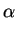. Csieq (2.44)

where0.44. This corresponds with the fact that under equilibrium conditions also

 Csieq = m . Coxnew (2.45)

must be fulfilled causing m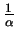. Under normal circumstances this is not true. Depending on the species different ratios between m andhave been found (Fig. 2.5):

• Boron (m): to the flux Fi via the interface the flux Fc due to silicon consumption is superpositioned and intensified. Thus boron tends to move towards the oxide layer and declines in the silicon layer.
• Arsenic and phosphorus (m > >): in contrast to boron the dopant concentration of arsenic and phosphorus is increasing at the silicon interface and a typical pile-up can be observed.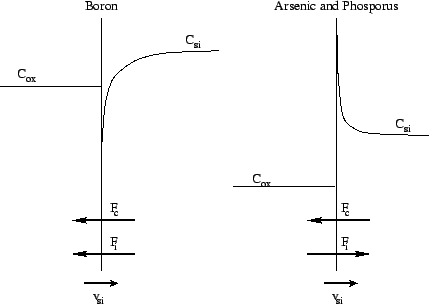The following general rules can be estimated for the segregation effect during an oxidizing process:
• the segregation effect increases in case of lower temperatures since the oxidation velocity decreases slower than the diffusion coefficients do.
• the higher the diffusion coefficient of a species in the silicon layer the faster non equilibrium states can be reduced, but the farther reaches the effect into the layer.
• wet oxidation favors the segregation effect because of higher oxidation velocity.Next:3. Numerical Discretization MethodsUp:2.2 OxidationPrevious:2.2.2 Point Defect Models
Mustafa Radi

1998-12-11